# 兔年了，利用AI風格化實現剪紙兔、年畫兔、煙花兔

## 一、影象風格化簡介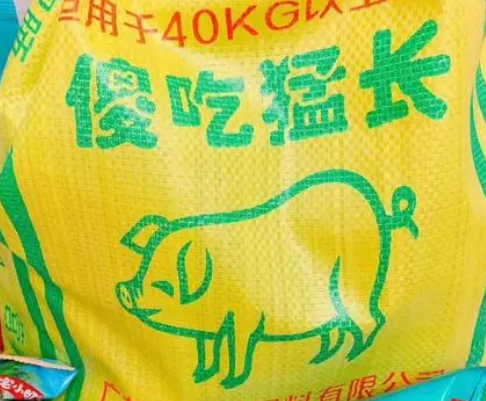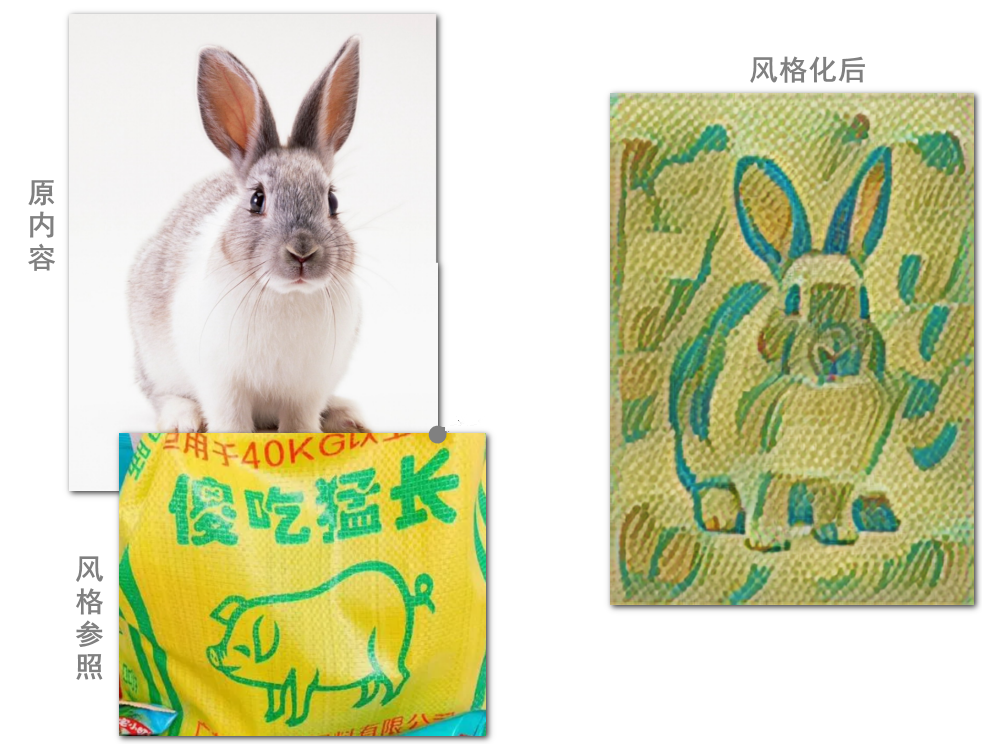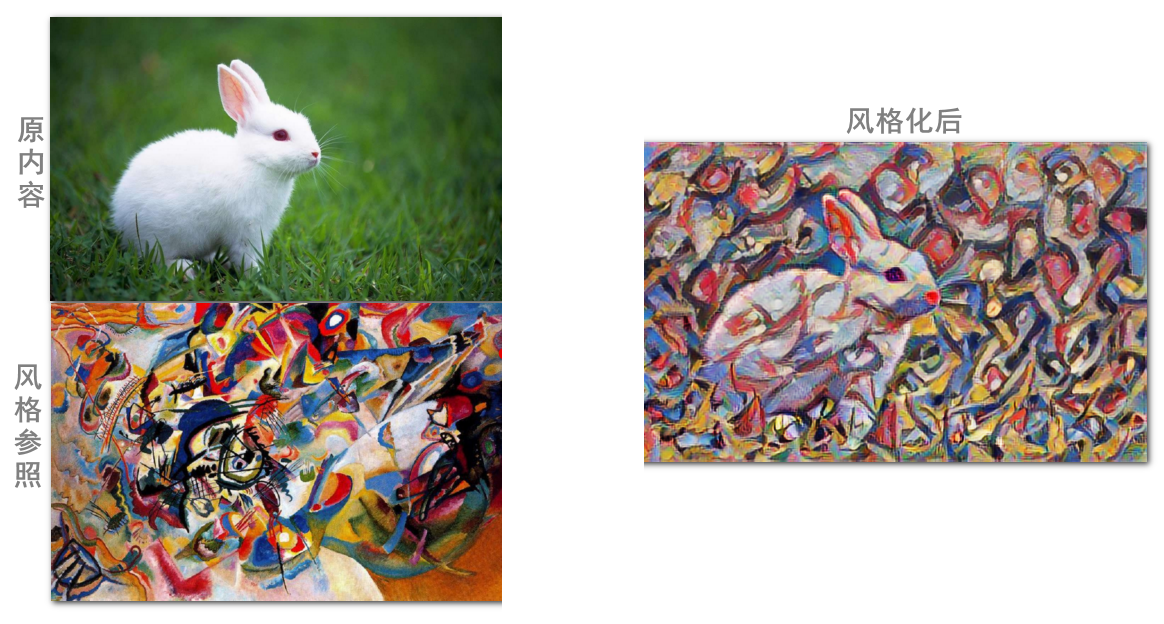## 二、技術實現講解

### 2.1 TensorFlow Hub庫

`TensorFlow` 已經很簡單和人性化了。簡單到幾十行程式碼，就可以實現數字識別的全流程（我都不好意寫這個教程）。

`Hub` 是輪轂的意思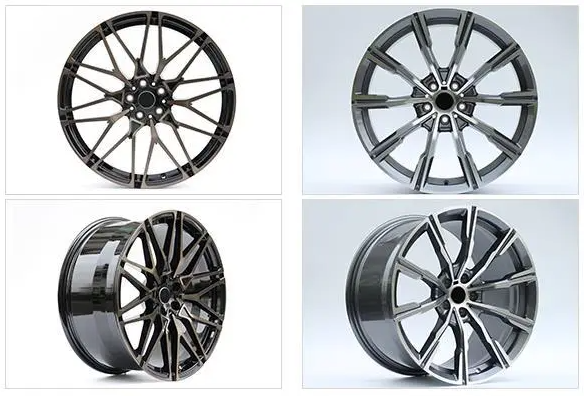### 2.2 載入image-stylization模型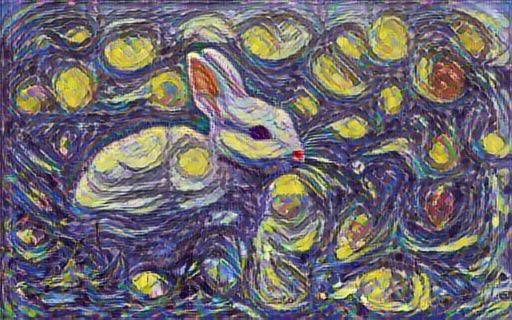``` python

# 匯入hub庫

import tensorflow_hub as hub

# 將content和style傳入

stylized_image = hub_model(content_image, style_image)

# 獲取風格化後的圖片並打印出來

tensor_to_image(stylized_image) ```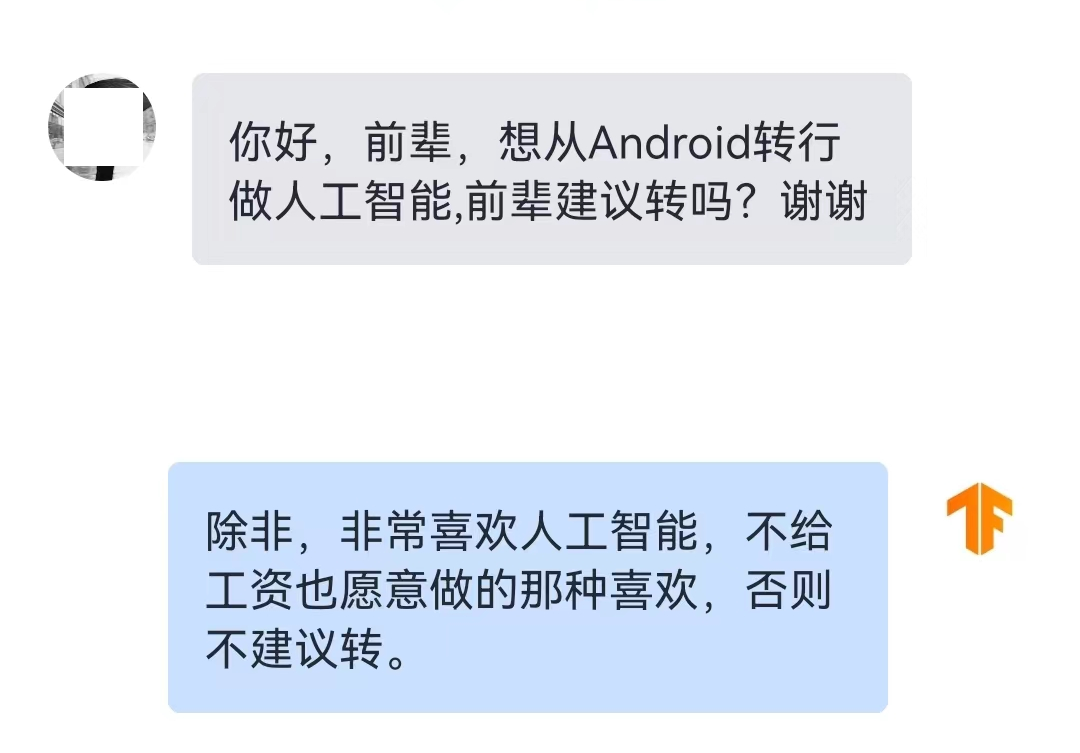### 2.3 輸入圖片轉為tensor格式

`hub_model = hub.load(……)` 是載入模型。我們是載入了影象風格化的模型。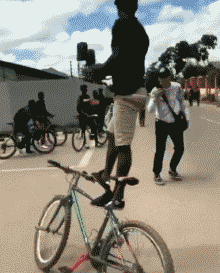`TensorFlow` 中的 `flow` 是什麼？這很像《道德經》裡的“道”是什麼一樣。它們只能在自己的語言體系裡能說清楚。

``` python import tensorflow as tf

# 根據路徑載入圖片，並縮小至512畫素，轉為tensor

max_dim = 512 img = tf.io.read_file(path_to_img) img = tf.image.decode_image(img, channels=3) img = tf.image.convert_image_dtype(img, tf.float32) shape = tf.cast(tf.shape(img)[:-1], tf.float32) long_dim = max(shape) scale = max_dim / long_dim new_shape = tf.cast(shape * scale, tf.int32) img = tf.image.resize(img, new_shape) img = img[tf.newaxis, :] tf_img = tf.constant(img) ```

`read_file` 從路徑讀入檔案。然後通過 `decode_image` 將檔案解析成陣列。

```python shape=(434, 650, 3), dtype=uint8 array([[[219, 238, 245], ..., [219, 238, 245]], [[219, 238, 245], ..., [219, 238, 245]]])```

`shape=(434, 650, 3)` 說明這是一個三維陣列，看資料這是一張650×434畫素且具有RGB三個通道的圖片。其中的array是具體畫素的數值，在某個顏色通道內，255表示純白，0表示純黑。

```python shape=(434, 650, 3), dtype=float32 array([[[0.8588236, 0.9333334, 0.9607844], ..., [0.8588236, 0.9333334, 0.9607844]], [[0.8588236, 0.9333334, 0.9607844], ..., [0.8588236, 0.9333334, 0.9607844]]])```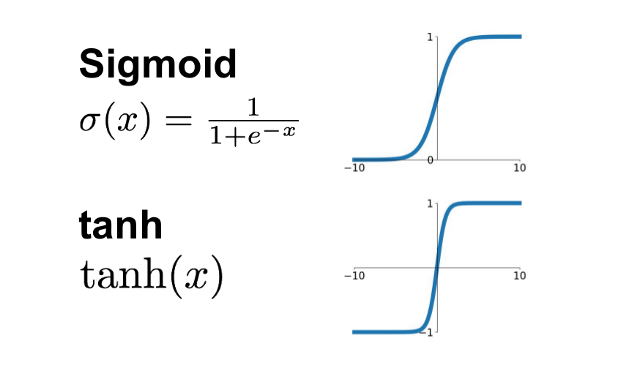```python shape=(341, 512, 3), dtype=float32 array([[[0.8588236, 0.9333334, 0.9607844], ..., [0.8588236, 0.9333334, 0.9607844]], [[0.8588236, 0.9333334, 0.9607844], ..., [0.8588236, 0.9333334, 0.9607844]]])```

`(434, 650, 3)` 代表的是一張圖。但是縱觀所有演算法模型，不管是 `model.fit(train_ds)` 訓練階段，還是 `model.predict(tf_imgs)` 預測階段。就沒有處理單張圖片的程式碼邏輯，全都是批量處理。

```python shape=(1, 341, 512, 3), dtype=float32 array([[[[0.8588236, 0.9333334, 0.9607844], ..., [0.8588236, 0.9333334, 0.9607844]], [[0.8588236, 0.9333334, 0.9607844], ..., [0.8588236, 0.9333334, 0.9607844]]]])```

`shape=(1, 341, 512, 3)` 表示有1張512×341的彩圖。那麼，這個結構它也可以承載100張這樣的圖，那時就是`shape=(100, 341, 512, 3)`。這就做到了，以不變應萬變。

### 2.4 tensor格式結果轉為圖片

```python [<tf.Tensor: shape=(1, 320, 512, 3), dtype=float32, numpy= array([[[[0.31562978, 0.47748038, 0.7790847 ], ..., [0.7430198 , 0.733053 , 0.6921962 ]], [[0.76158 , 0.6912774 , 0.5468565 ], ..., [0.69527835, 0.70888966, 0.6492392 ]]]], dtype=float32)>]```

``` python import numpy as np import PIL.Image

tensor = stylized_images tensor = tensor*255 tensor_arr = np.array(tensor, dtype=np.uint8) img_arr = tensor_arr img = PIL.Image.fromarray(img_arr) img.save(n_path) ```

• 第1步：取結果中的第一個 `stylized_images` ，那是 `shape=(1, 320, 512, 3)`
• 第2步：小數轉為255色值的整數陣列 `tensor*255``np.array(tensor, dtype=np.uint8)`
• 第3步：取出 `shape=(1, 320, 512, 3)` 中的那個1，也就是512×320的那張圖。
• 第4步：通過 `fromarray(img_arr)` 載入圖片的陣列資料，儲存為圖片檔案。

## 三、一切皆可兔圖的效果

### 3.1 年畫兔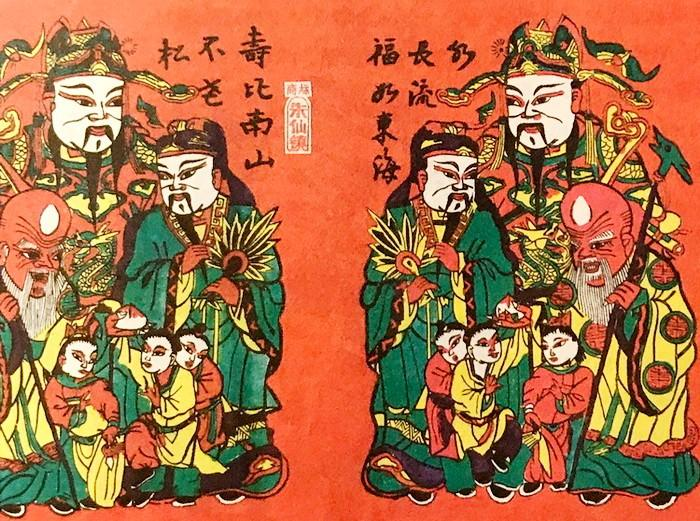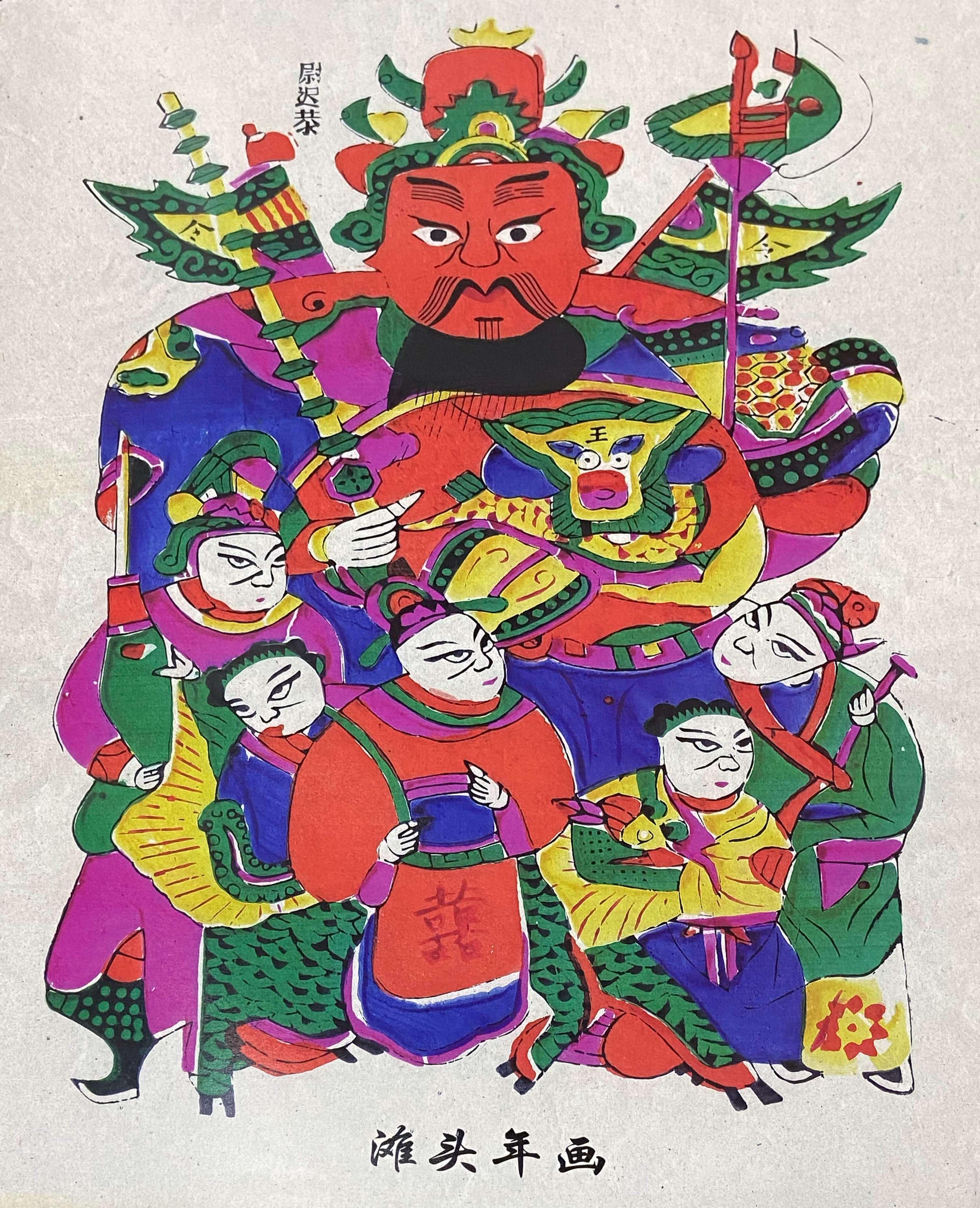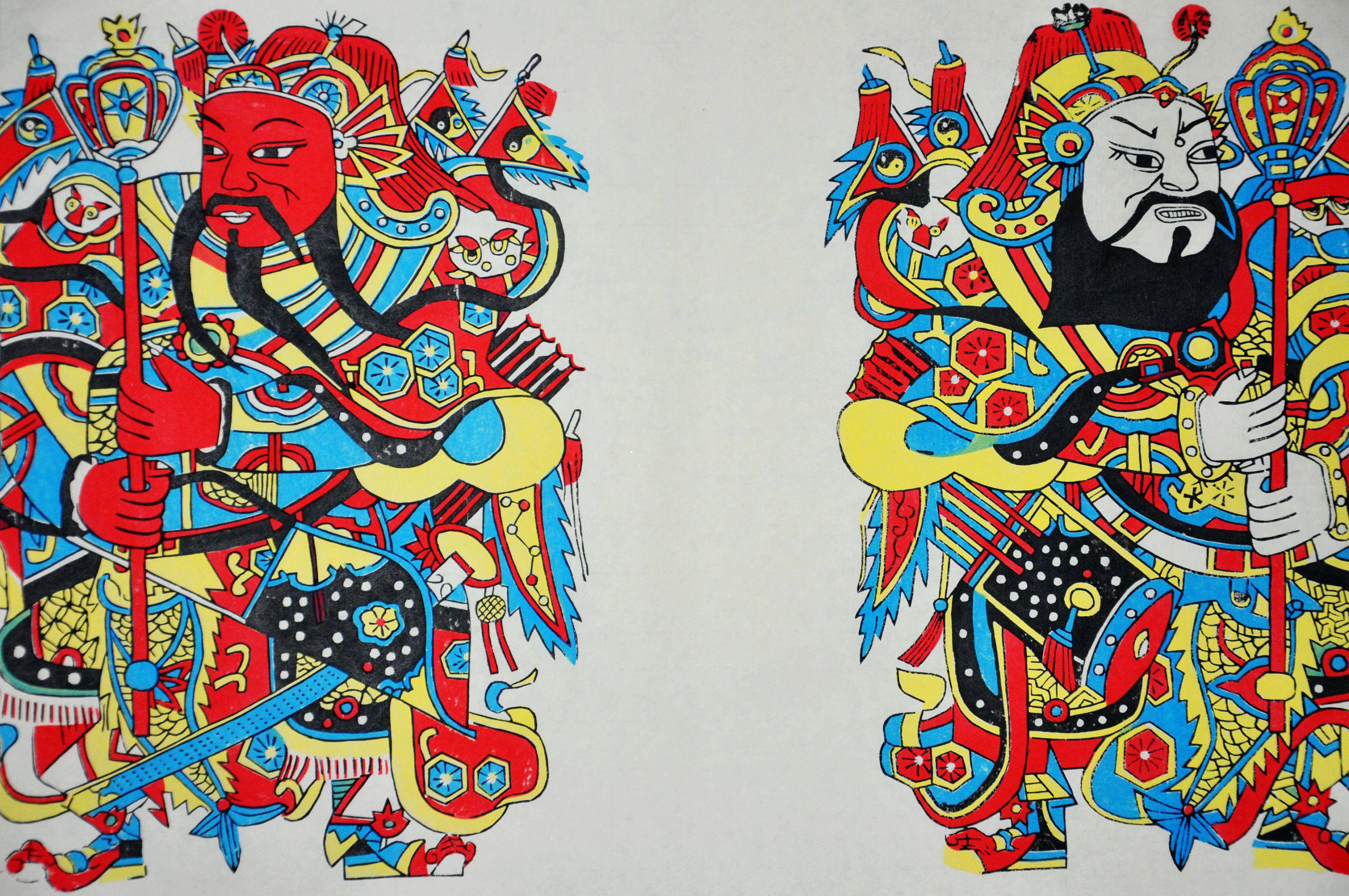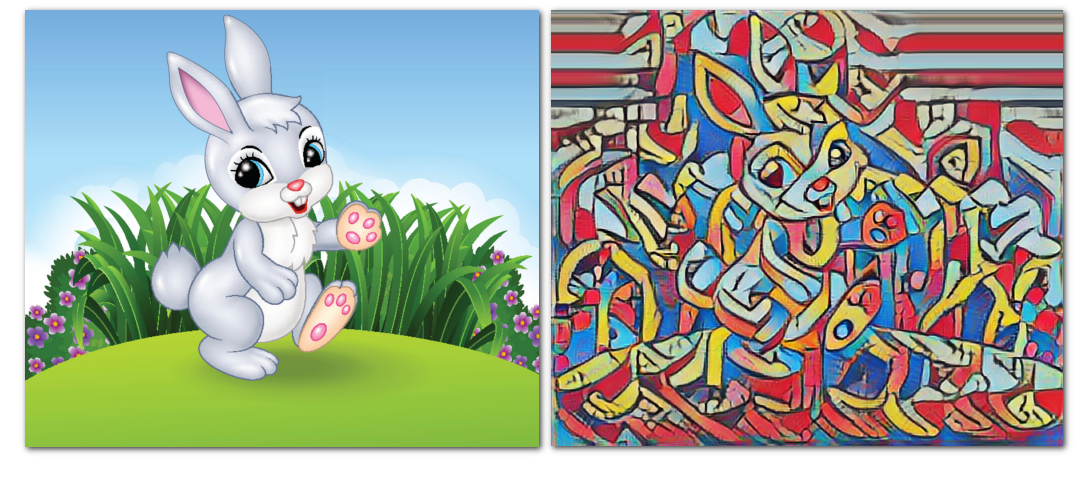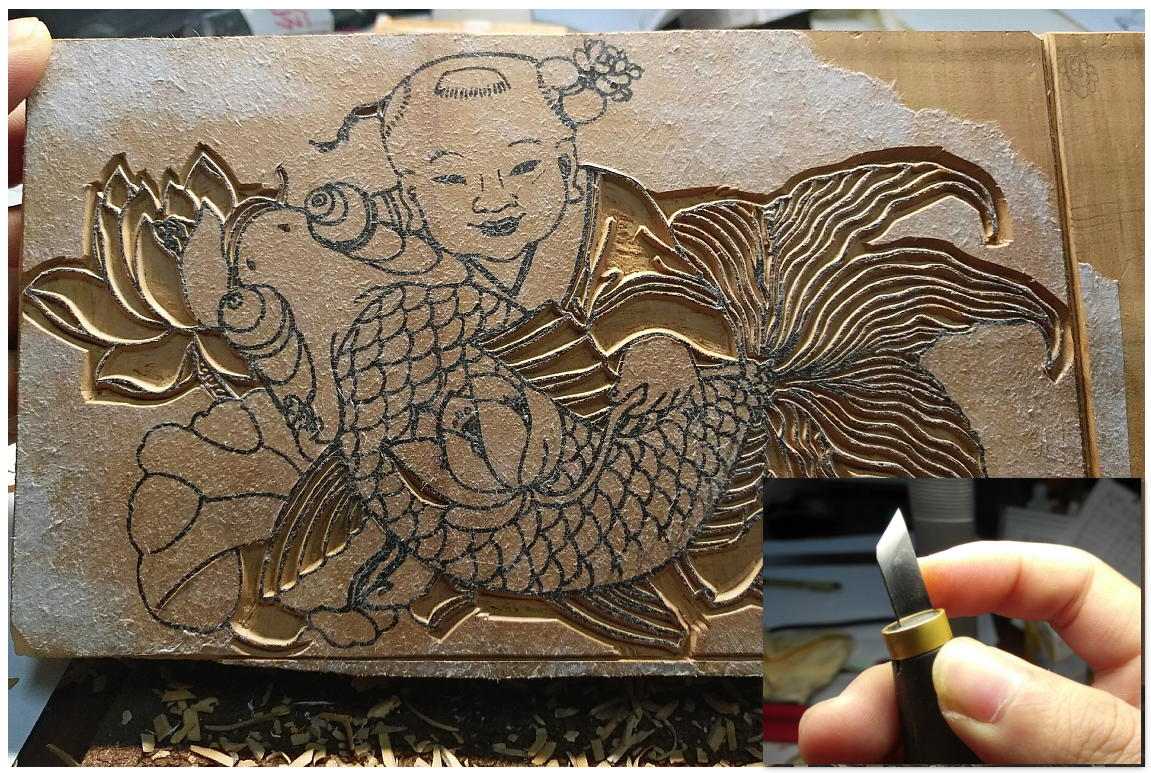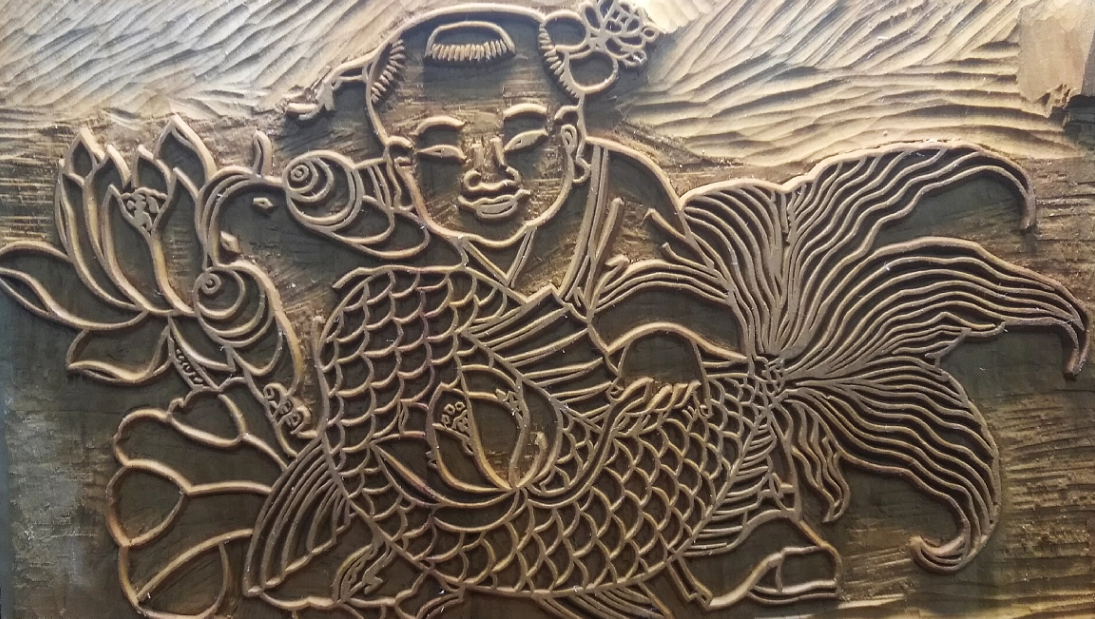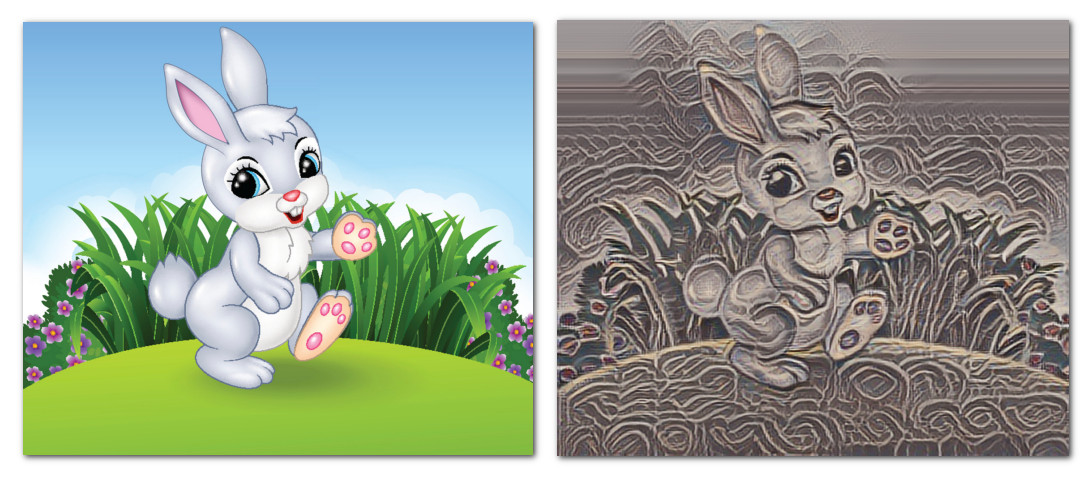### 3.2 剪紙兔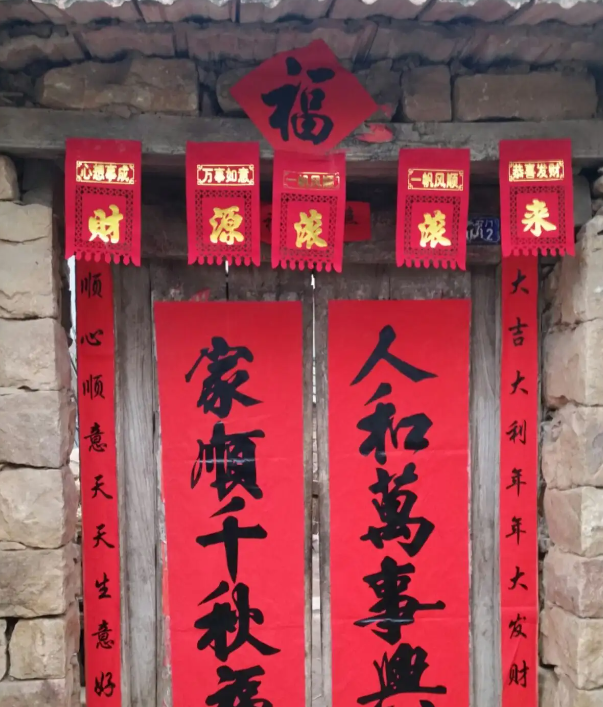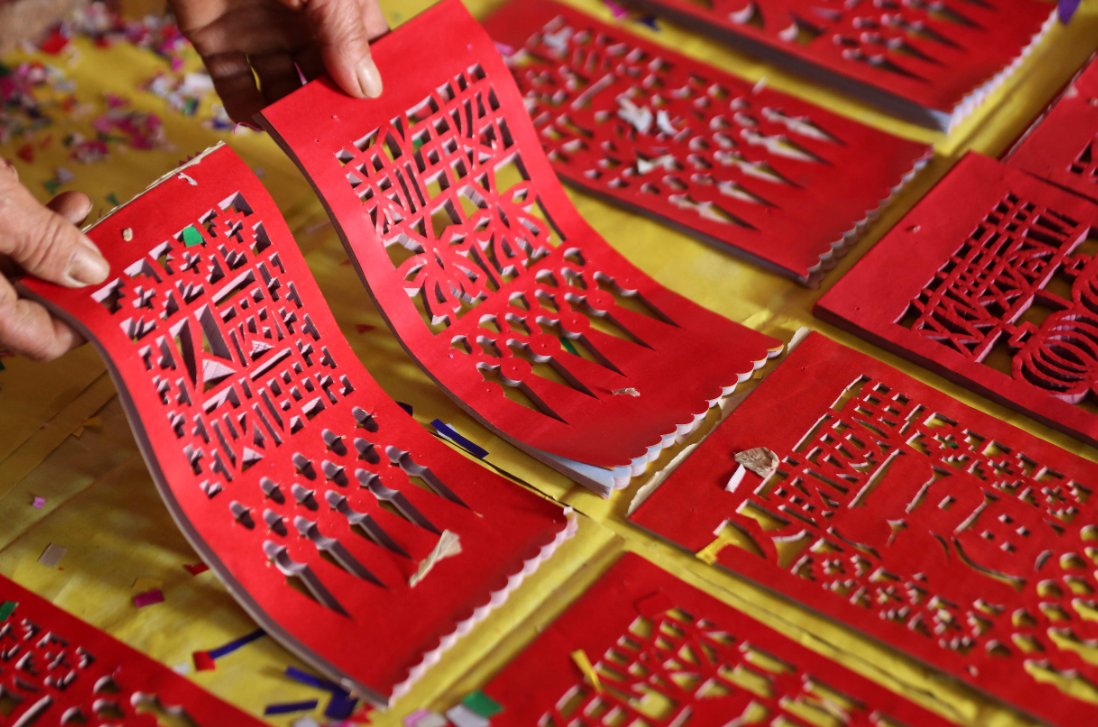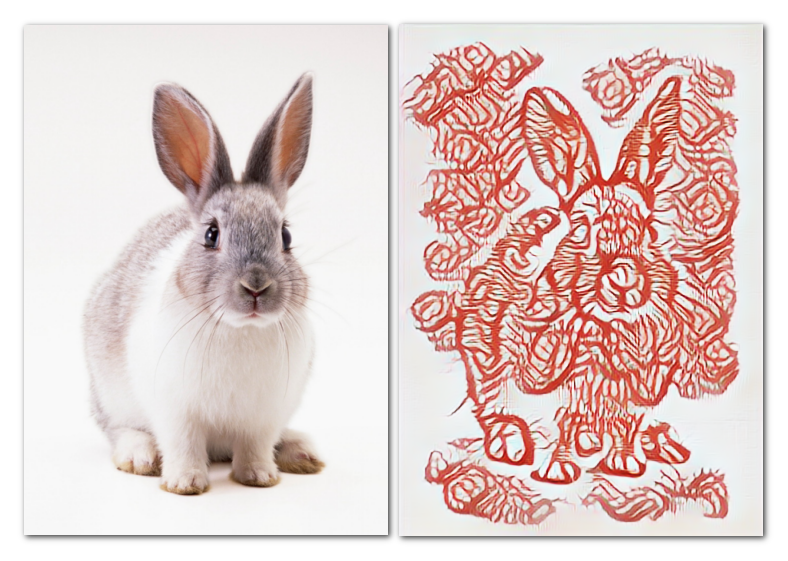### 3.3 煙花兔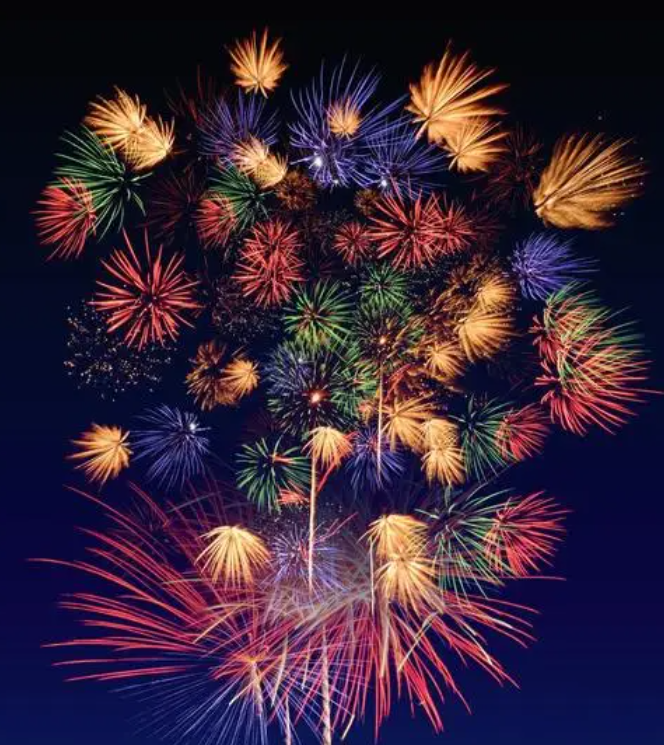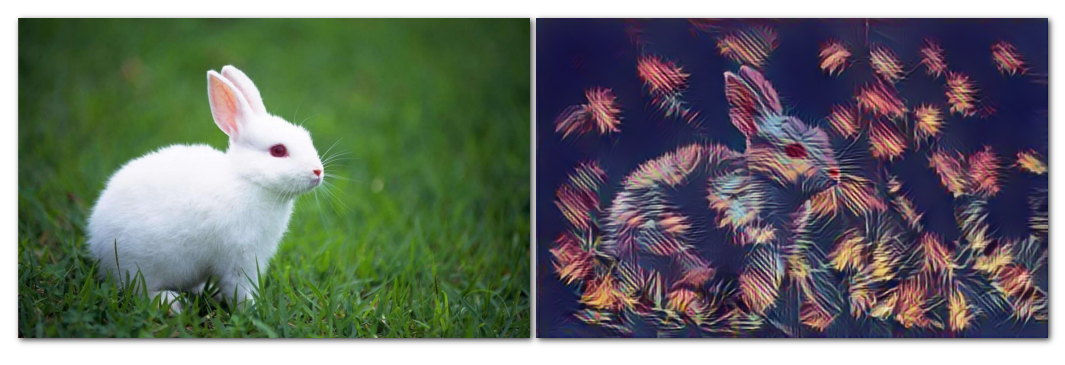## 四、無限遐想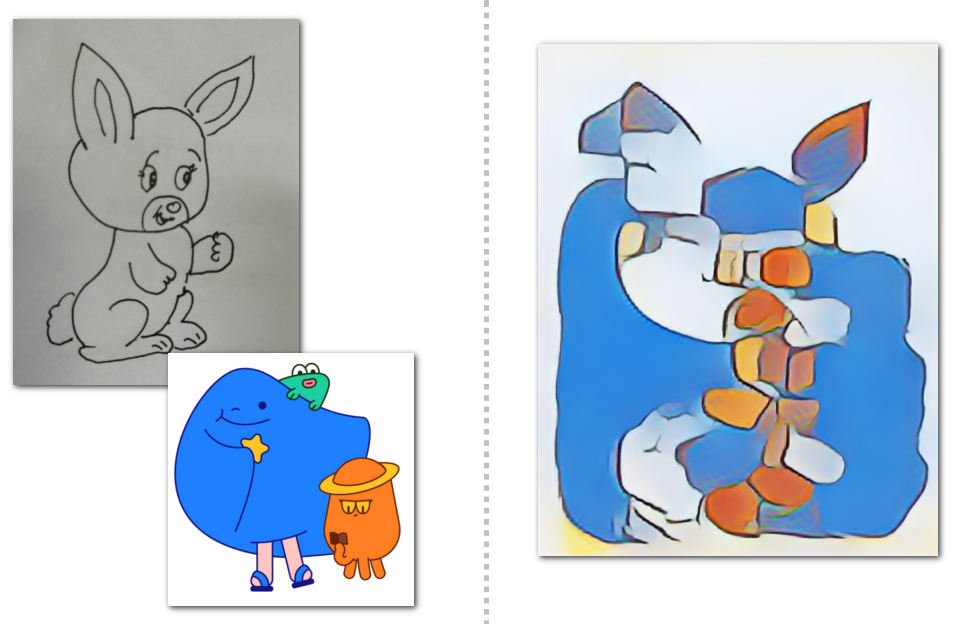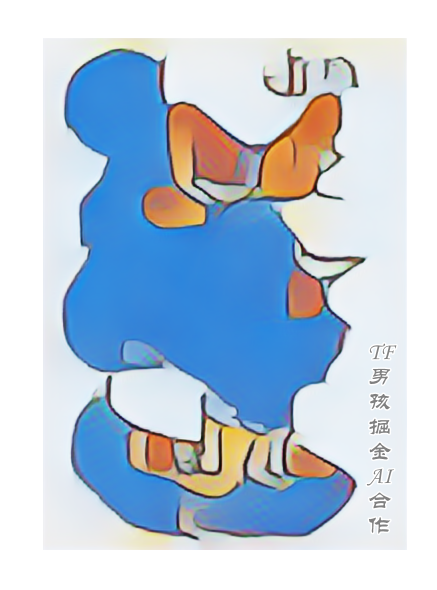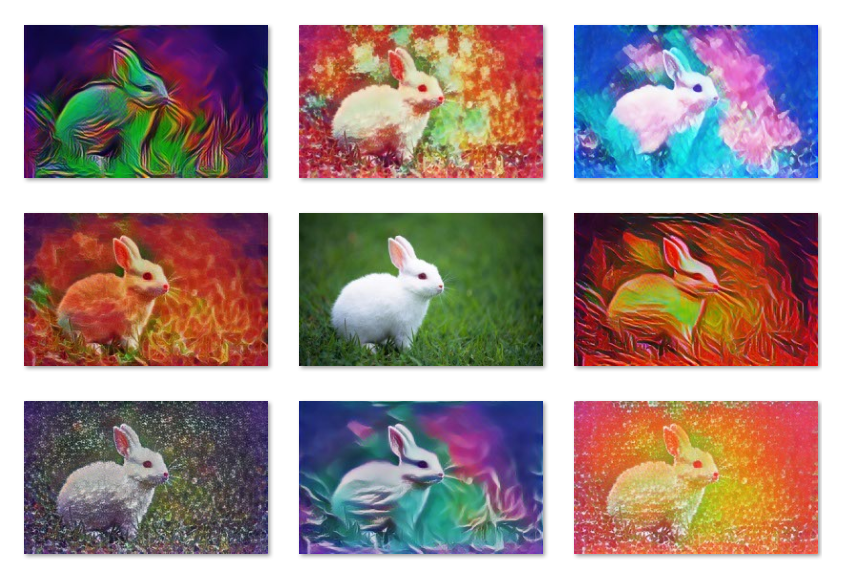「其他文章」